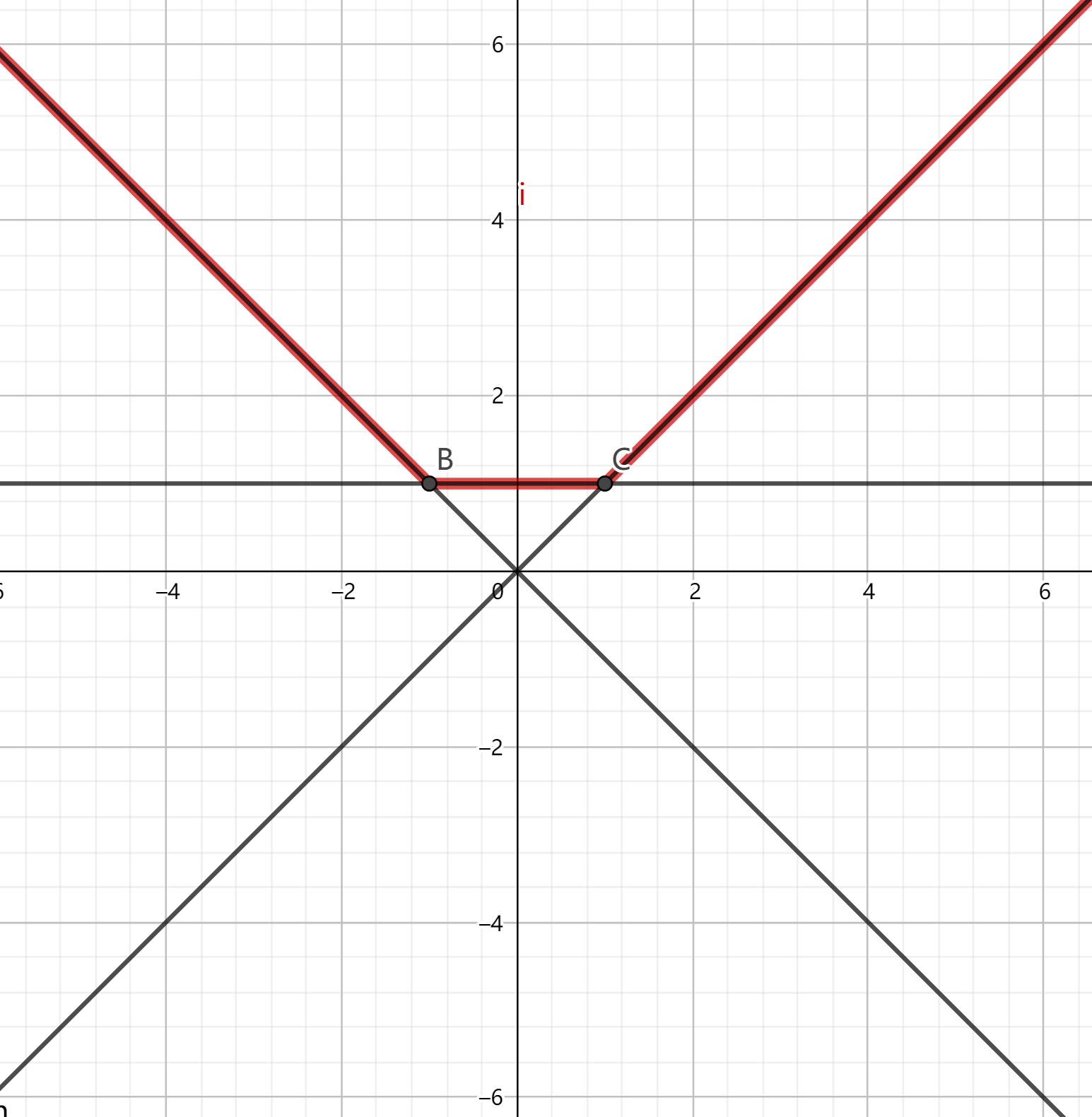# 凸包技巧

• 向集合插入一个一阶多项式。
• 询问集合中所有一阶多项式在某个特定$x=x_0$值处的值的最大值。

$f(x)=\max_{y\in Y}y(x)$# 单调增斜率优化

$f(i)=\Bigg\{ \begin{array}{ccc} 0&,i=0\\ \min\{h(j)-s(i)s(j)|0\leq j <i\}&,i\ge 1 \end{array}$

$r(j,i) \leq r(k,i)\\ \Rightarrow h(j)-s(i)s(j) \leq h(k)-s(i)s(k)\\ \Rightarrow h(j)-h(k) \leq s(i)(s(j)-s(k))\\ \Rightarrow \frac{h(j)-h(k)}{s(j)-s(k)}\leq s(i)$

$r(j,i) \leq r(k,i)\\ \Rightarrow \frac{Y(j)-Y(k)}{X(j)-X(k)} \leq s(i)$

SRM787 div1第一题。